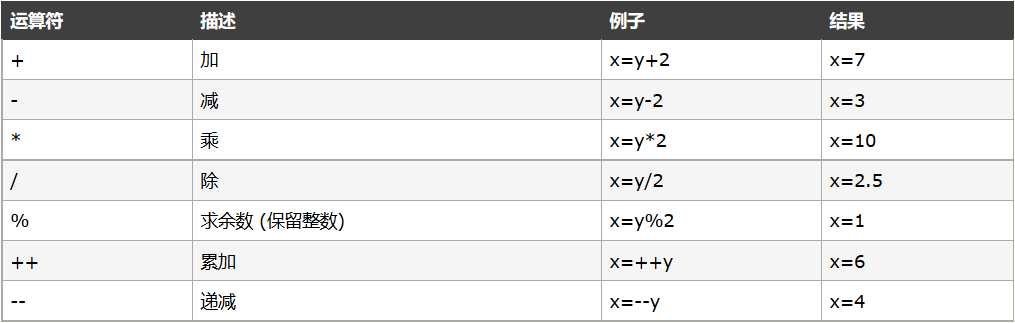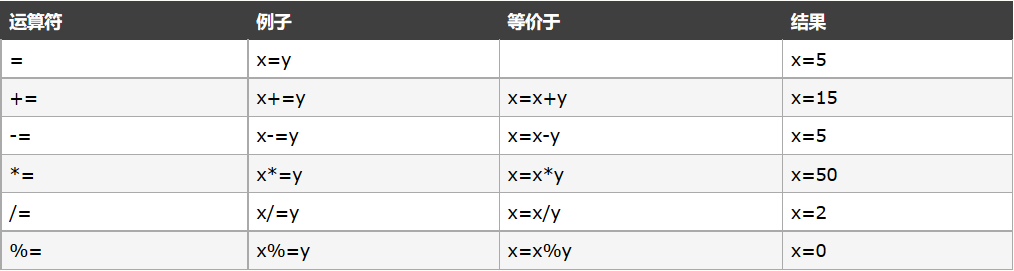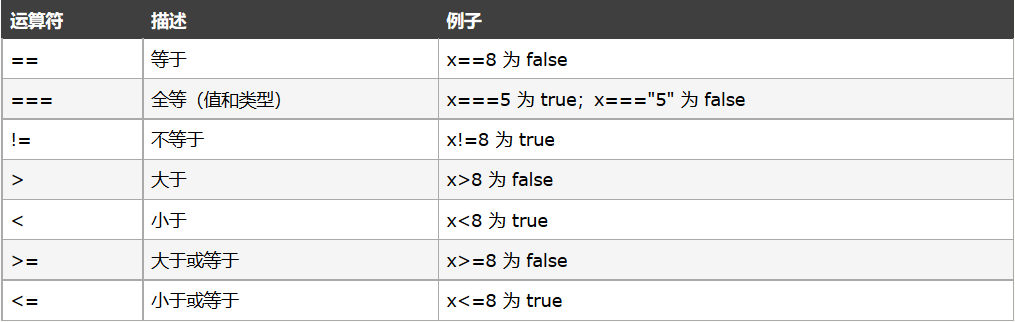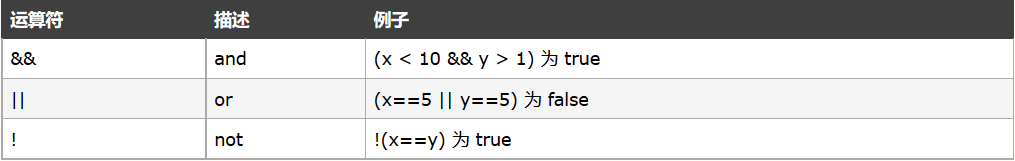JavaScript基础学习笔记

JavaScript 是脚本语言

JavaScript 是一种轻量级的编程语言。

JavaScript 是可插入 HTML 页面的编程代码。

JavaScript 插入 HTML 页面后，可由所有的现代浏览器执行。

一些主要的内容：

写入 HTML 输出：

<!DOCTYPE html>
<html>
<body>
<p>JavaScript 能够直接写入 HTML 输出流中：</p>
<script>
document.write("<h1>这是h1标签</h1>");
document.write("<p>这是p标签</p>");
</script>
</body>
</html>

对事件作出反应：

<!DOCTYPE html>
<html>
<body>
<h1>对事件作出反应</h1>
<p>单击按钮弹出welcome</p>
</body>
</html>

改变html内容：

<!DOCTYPE html>
<html>
<body>
<h1>我的第一段 JavaScript</h1>
<p id="demo">JavaScript 能改变 HTML 元素的内容。</p>
<script>
function myFunction() {    //定义一个函数
x = document.getElementById("demo");  // 找到元素（用getElementById获取页面id=demo的元素）
x.innerHTML = "Hello JavaScript!";    // 改变内容
}
</script>
<button type="button" onclick="myFunction()">点击这里</button>    <!--当点击按钮时，执行myFunction函数-->
</body>
</html>

改变HTML样式：

<!DOCTYPE html>
<html>
<body>
<h1>改变html样式</h1>
<p id="demo">更改样式之前</p>    <!--更改样式之前默认为黑色字体-->
<script>
function myFunction() {
x = document.getElementById("demo") // 找到元素
x.style.color = "#ff0000";          // 改变样式（改变样式之后为红色：#ff0000=红色）
}
</script>
<button type="button" onclick="myFunction()">点击这里</button>
</body>
</html>

验证输入：

<!DOCTYPE html>
<html>
<body>
<h1>验证输入的内容是否为number</h1>
<p>请输入数字。如果输入值不是数字，浏览器会弹出提示框。</p>
<input id="demo" type="text">   <!--文本类型，输入框-->
<script>
function myFunction() {
var x = document.getElementById("demo").value;    //获取id的值，赋给x
if (x == "" || isNaN(x)) {    //判断：如果没有输入，或者非数字类型，及弹出Not Numeric
}
}
</script>
<button type="button" onclick="myFunction()">点击这里</button>
</body>
</html>

JavaScript的使用：

HTML 中的脚本必须位于 <script> 与 </script> 标签之间。脚本可被放置在 HTML 页面的 <body> 和 <head> 部分中。

<script> 标签：

<script> 和 </script> 之间的代码行包含了 JavaScript：

<script>
</script>

<body> 中的 JavaScript：

JavaScript 会在页面加载时向 HTML 的 <body> 写文本：

<!DOCTYPE html>
<html>
<body>
<p>JavaScript 能够直接写入 HTML 输出流中：</p>
<script>
document.write("<h1>这是h1标签</h1>");
document.write("<p>这是p标签</p>");
</script>
</body>
</html>

<head> 中的 JavaScript 函数：

<!DOCTYPE html>
<html>
<script>
function myFunction() {
document.getElementById("demo").innerHTML = "变了吧！！！";    //获取id=demo的标签，改变内容
}
</script>
<body>
<p id="demo">点击下面按钮会变哟！</p>
<button type="button" onclick="myFunction()">点击这里</button>  <!--单击执行函数-->
</body>
</html>

<body> 中的 JavaScript 函数：

<!DOCTYPE html>
<html>
<body>
<p id="demo">单击此下面按钮会变哟！</p>
<button type="button" onclick="myFunction()">点击这里</button>
<script>
function myFunction() {
document.getElementById("demo").innerHTML = "变了吧！！！";
}
</script>
</body>
</html>

外部的 JavaScript：

.
├── index.html
├── js
|   ├── myScript.js
└── css
<!DOCTYPE html>
<html>
<body>
<script src="js/myScript.js"></script>　　//导入js目录下的myScript.js文件
</body>
</html>

JavaScript输出：

JavaScript 通常用于操作 HTML 元素。

操作 HTML 元素

<!DOCTYPE html>
<html>
<body>
<p id="demo"></p>    <!--里面没有任何内容-->
<p id="demo1">123</p>    <!--会将123替换为456-->
<script>
document.getElementById("demo").innerHTML = "JavaScript";    //获取demo，里面没有任何内容，然后我们用（innerHTML）替换为 "JavaScript"。
document.getElementById("demo1").innerHTML = "456";     //获取demo1，用innerHTML替换为456
</script>
</body>
</html>

警告

<!DOCTYPE html>
<html>
<body>
<h1>123</h1>
<p>456</p>
<button onclick="myFunction()">点击这里</button>    <!--当点击时，会执行myFunction()函数，但是函数只执行了document.write(),所以整个网页就直接不覆盖了-->
<script>
function myFunction() {
document.write("糟糕！文档消失了。");
}
</script>
</body>
</html>

JavaScript 代码块

JavaScript 语句通过代码块的形式进行组合。

JavaScript 函数是将语句组合在块中的典型例子。

<!DOCTYPE html>
<html>
<body>
<p id="myPar"></p>
<div id="myDiv"></div>
<p><button type="button" onclick="myFunction()">点击这里</button></p>    <!--点击时执行函数-->
<script>
function myFunction() {
document.getElementById("myPar").innerHTML = "Hello World";    //向 id="myPar"的HTML元素输出文本 "Hello World"
document.getElementById("myDiv").innerHTML = "How are you?";
}
</script>
</body>
</html>

JavaScript 对大小写敏感。

JavaScript 对大小写是敏感的。

// 这是单行注释

/*

*/

变量是储存的容器：

var x = 2;
var y = 3;
var z = x + y;
document.write(x,",",y,",",z);

JavaScript 语句和 JavaScript 变量都对大小写敏感。

声明（创建） JavaScript 变量

var carname;

变量声明之后，该变量是空的（它没有值）。

carname = "Volvo";

当然，你也可以在声明变量时对其赋值：

var carname = "Volvo";

<button onclick="myFunction()">点击这里</button>
<p id="demo"></p>
<script>
function myFunction() {
var carname = "Volvo";
document.getElementById("demo").innerHTML = carname;
}
</script>

一条语句，声明多个变量：

var name = "smelond", age = 16, job = "Student";

当然，也可以横跨多行：

var name = "smelond",
age = 16,
job = "Student";

var carname;

重新声明JavaScript变量：

var carname = "Volvo";
var carname;

字符串、数字、布尔、数组、对象、Null、Undefined

JavaScript 拥有动态类型

JavaScript 拥有动态类型。这意味着相同的变量可用作不同的类型：

var x                // x 为 undefined
var x = 6;           // x 为数字
var x = "Bill";      // x 为字符串

JavaScript 字符串

var carname1="Bill Gates";
var carname2='Bill Gates';
var answer1="Nice to meet you!";
var answer2="He is called 'Bill'";
var answer3='He is called "Bill"';

document.write(carname1 + "<br>")    //Bill Gates
document.write(carname2 + "<br>")    //Bill Gates
document.write(answer1 + "<br>")     //Nice to meet you!
document.write(answer2 + "<br>")     //He is called 'Bill'
document.write(answer3 + "<br>")     //He is called "Bill"

JavaScript 数字

JavaScript 只有一种数字类型。数字可以带小数点，也可以不带：

var x1=36.00;
var x2=36;
var y=123e5;
var z=123e-5;

document.write(x1 + "<br />")    //36
document.write(x2 + "<br />")    //36
document.write(y + "<br />")     //12300000
document.write(z + "<br />")     //0.00123

JavaScript 数组：

var cars = new Array();
cars = "Audi";
cars = "BMW";
cars = "Volvo";

var cars = new Array("Audi", "BWM", "Volvo");

var cars = new Array();
cars = "Audi";
cars = "BMW";
cars = "Volvo";
for (var i=0;i<cars.length;i++){    //cars = 3,条件是i不能等于3，所以循环3次，刚好将数组里面的内容循环完成
document.write(cars[i] + "<br>");    //每次循环输出cars,cars就表示Audi，表示BMW。。。
}

JavaScript 对象

var person = {username:"smelond", age:16, id:1};

var person = {
age         : 16,
id            : 1
};

实例：

var person = {username:"smelond", age:16, id:1};
document.write(person.username+ "<br />");     //smelond
document.write(person["username"] + "<br />");     //smelond
document.write(person.age+ "<br />");     //16
document.write(person["age"] + "<br />");     //16
document.write(person.id+ "<br />");     //1
document.write(person["id"] + "<br />");     //1

Undefined 和 Null

Undefined 这个值表示变量不含有值。

var person;
var car="Volvo";
document.write(person + "<br />");     //undefined
document.write(car + "<br />");     //Volvo
var car=null
document.write(car + "<br />");     //null

声明变量类型

JavaScript 变量均为对象。当您声明一个变量时，就创建了一个新的对象。

var carname=new String;
var x=      new Number;
var y=      new Boolean;
var cars=   new Array;
var person= new Object;

函数是由事件驱动的或者当它被调用时执行的可重复使用的代码块。

JavaScript 函数语法

function functionname() {
执行的代码
}

调用带参数的函数

functionname(argument1,argument2)

function myFunction(var1,var2) {
这里是要执行的代码
}

例子：

<button onclick="myFunction('smelond','16')">点击这里</button>
<script>
function myFunction(name,age){
alert("你好:" + name + "\n" + "你的年龄：" + age);
}
</script>

上面的函数会当按钮被点击时提示：

带有返回值的函数

function myFunction(){
var x=5;
return x;
}

var myVar=myFunction();

myVar 变量的值是 5，也就是函数 "myFunction()" 所返回的值。

document.getElementById("demo").innerHTML=myFunction();

"demo" 元素的 innerHTML 将成为 5，也就是函数 "myFunction()" 所返回的值。

function myFunction(a,b) {
return a*b;
}
document.getElementById("demo").innerHTML=myFunction(4,3);

demo元素的innerHTML的值将是：12

function myFunction(a,b){
if (a>b){
return;
}
x=a+b
}

如果 a 大于 b，则上面的代码将退出函数，并不会计算 a 和 b 的总和。

JavaScript 变量的生存期

JavaScript 变量的生命期从它们被声明的时间开始。

carname="Volvo";

JavaScript 运算符

JavaScript 算术运算符JavaScript 赋值运算符用于字符串的 + 运算符

+ 运算符用于把文本值或字符串变量加起来（连接起来）。

txt1="What a very";
txt2="nice day";
txt3=txt1+txt2;

txt1="What a very ";
txt2="nice day";
txt3=txt1+txt2;

txt1="What a very";
txt2="nice day";
txt3=txt1+" "+txt2;

"What a very nice day"

x=5+5;
document.write(x);
document.write("<br />");     //10
x="5"+"5";
document.write(x);
document.write("<br />");     //55
x=5+"5";
document.write(x);
document.write("<br />");     //55
x="5"+5;
document.write(x);
document.write("<br />");     //55

比较运算符如何使用

if (age<16) document.write("Too young");

逻辑运算符条件运算符

JavaScript 还包含了基于某些条件对变量进行赋值的条件运算符。

语法

variablename=(condition)?value1:value2

例子

greeting=(visitor=="PRES")?"Dear President ":"Dear ";

JavaScript If...else 语句:

条件语句

• if 语句 - 只有当指定条件为 true 时，使用该语句来执行代码
• if...else 语句 - 当条件为 true 时执行代码，当条件为 false 时执行其他代码
• if...else if....else 语句 - 使用该语句来选择多个代码块之一来执行
• switch 语句 - 使用该语句来选择多个代码块之一来执行

语法

if (条件){
只有当条件为 true 时执行的代码
}

实例

<button onclick="myFunction()">点击这里</button>
<p id="demo"></p>
<script>
function myFunction() {
var x = "";
var time = new Date().getHours();    //获取当前小时时间，24小时制
if (time < 20)    //如果当前时间大于20点，将Good day赋值给x
{
x = "Good day";
}
document.getElementById("demo").innerHTML = x;
}
</script>

语法

if (条件){
当条件为 true 时执行的代码
}else{
当条件不为 true 时执行的代码
}

<button onclick="myFunction()">点击这里</button>
<p id="demo"></p>
<script>
function myFunction(){
var x="";
var time=new Date().getHours();
if (time<20){
x="Good day";
}else{    //如果不大于20，就将Good evening赋给x
x="Good evening";
}
document.getElementById("demo").innerHTML=x;
}
</script>

If...else if...else 语句

语法

if (条件 1){
当条件 1 为 true 时执行的代码
}
else if (条件 2){
当条件 2 为 true 时执行的代码
}
else{
当条件 1 和 条件 2 都不为 true 时执行的代码
}

Switch 语句

switch 语句用于基于不同的条件来执行不同的动作。

语法

switch(n){
case 1:
执行代码块 1
break;
case 2:
执行代码块 2
break;
default:
n 与 case 1 和 case 2 不同时执行的代码
}

<!DOCTYPE html>
<html>
<body>
<button onclick="myFunction()">点击这里</button>
<p id="demo"></p>
<script>
function myFunction() {
var x;
var d = new Date().getDay();    //返回星期中的某一天
switch (d) {
case 0:
x = "Today it's Sunday";
break;
case 1:
x = "Today it's Monday";
break;
case 2:
x = "Today it's Tuesday";
break;
case 3:
x = "Today it's Wednesday";
break;
case 4:
x = "Today it's Thursday";
break;
case 5:
x = "Today it's Friday";
break;
case 6:
x = "Today it's Saturday";
break;
}
document.getElementById("demo").innerHTML = x;
}
</script>

</body>
</html>

实例

<html>
<body>
<button onclick="myFunction()">点击这里</button>
<p id="demo"></p>
<script>
function myFunction() {
var x;
var d = new Date().getDay();    //今天星期一，所以d=1
switch (d) {
case 6:    //d!=6
x = "Today it's Saturday";
break;
case 0:    //d!=0
x = "Today it's Sunday";
break;
default:    //所以就给了一个default
x = "Looking forward to the Weekend";
}
document.getElementById("demo").innerHTML = x;
}
</script>
</body>
</html>

For 循环

JavaScript 循环

document.write(cars + "<br>");
document.write(cars + "<br>");
document.write(cars + "<br>");
document.write(cars + "<br>");
document.write(cars + "<br>");
document.write(cars + "<br>");

for (var i=0;i<cars.length;i++) {
document.write(cars[i] + "<br>");
}

不同类型的循环

JavaScript 支持不同类型的循环：

• for - 循环代码块一定的次数
• for/in - 循环遍历对象的属性
• while - 当指定的条件为 true 时循环指定的代码块
• do/while - 同样当指定的条件为 true 时循环指定的代码块

For 循环

for 循环是您在希望创建循环时常会用到的工具。

for (语句 1; 语句 2; 语句 3) {
被执行的代码块
}

<button onclick="myFunction()">点击这里</button>
<p id="demo"></p>
<script>
function myFunction() {
var x = "";
for (var i = 0; i < 5; i++) {
x = x + "The number is " + i + "<br>";
}
document.getElementById("demo").innerHTML = x;
}
</script>

语句 1

实例:

<script>
cars = ["BMW", "Volvo", "Saab", "Ford"];        //数组
for (var i = 0, j = cars.length; i < j; i++) {　　//j = 4,如果i<j，name执行for循环，从0开始执行
document.write(cars[i] + "<br>");
}
</script>

<script>
cars = ["BMW", "Volvo", "Saab", "Ford"];
var i = 2, len = cars.length;
for (; i < len; i++) {
document.write(cars[i] + "<br>");
}
</script>

语句 3

<script>
cars = ["BMW", "Volvo", "Saab", "Ford"];
var i = 0, len = cars.length;
for (; i < len;) {
document.write(cars[i] + "<br>");
i++;    //当我执行第一次循环后i自加1，其实跟放到括号里面差不多
}
</script>

For/In 循环

JavaScript for/in 语句循环遍历对象的属性：

<button onclick="myFunction()">点击这里</button>
<p id="demo"></p>
<script>
function myFunction() {
var x;
var txt = "";
var person = {username: "smelond", age: "16", job: "Student"};    //对象
for (x in person) {    //用in遍历对象的属性
txt = txt + person[x] + "<br />";
}
document.getElementById("demo").innerHTML = txt;
}
</script>

While 循环

While 循环会在指定条件为真时循环执行代码块。

语法

while (条件){
需要执行的代码
}

<button onclick="myFunction()">点击这里</button>
<p id="demo"></p>
<script>
function myFunction() {
var x = "", i = 0;
while (i < 5) {
x = x + "The number is " + i + "<br>";
i++;
}
document.getElementById("demo").innerHTML = x;
}
</script>

do/while 循环

do/while 循环是 while 循环的变体。该循环会执行一次代码块，在检查条件是否为真之前，然后如果条件为真的话，就会重复这个循环。

语法

do{
需要执行的代码
}
while (条件);

<button onclick="myFunction()">点击这里</button>
<p id="demo"></p>
<script>
function myFunction() {
var x = "", i = 0;
do {    //会至少先循环一次
x = x + "The number is " + i + "<br>";
i++;    //现在的i=1了
}
while (i < 5)    //判断i是否小于5，如果等于5就不执行了，如果while里面写着i<0，当然我们刚刚已经执行了一次了，所以直接停止，并且执行了一次
document.getElementById("demo").innerHTML = x;
}
</script>

for 语句实例

<script>
cars = ["BMW", "Volvo", "Saab", "Ford"];
var i = 0;
for (; cars[i];) {
document.write(cars[i] + "<br>");
i++;
}
</script>

while 语句实例

<script>
cars = ["BMW", "Volvo", "Saab", "Ford"];
var i = 0;
while (cars[i]) {
document.write(cars[i] + "<br>");
i++;
}
</script>

Break 和 Continue 语句

break 语句用于跳出循环。

continue 用于跳过循环中的一个迭代。

Break 语句

break 语句可用于跳出循环。

break 语句跳出循环后，会继续执行该循环之后的代码（如果有的话）：

<button onclick="myFunction()">点击这里</button>
<p id="demo"></p>
<script>
function myFunction() {
var x = "";
for (i = 0; i < 10; i++) {
if (i == 3) {    //小于3都不会执行这个if语句，等于3后执行这个if，会直接跳出for循环
break;
}
x = x + "The number is " + i + "<br>";    //赋值3次
}
document.getElementById("demo").innerHTML = x;
}
</script>

if (i == 3) break;

Continue 语句

continue 语句中断循环中的迭代，如果出现了指定的条件，然后继续循环中的下一个迭代。

<button onclick="myFunction()">点击这里</button>
<p id="demo"></p>
<script>
function myFunction() {
var x = "", i = 0;
for (i = 0; i < 10; i++) {
if (i == 3) continue;    //如果i==3，则跳过，再次执行for循环，从4开始，这句直接省略了大括号{}，因为if语句只有一行代码
x = x + "The number is " + i + "<br>";
}
document.getElementById("demo").innerHTML = x;
}
</script>

JavaScript 标签

break 和 continue 语句仅仅是能够跳出代码块的语句。

语法

break labelname;

continue labelname;

continue 语句（带有或不带标签引用）只能用在循环中。

break 语句（不带标签引用），只能用在循环或 switch 中。

<script>
cars = ["BMW", "Volvo", "Saab", "Ford"];    //数组
list:
{
document.write(cars + "<br>");
document.write(cars + "<br>");
document.write(cars + "<br>");
break list;    //直接跳出这个代码块，
document.write(cars + "<br>");
document.write(cars + "<br>");    //如果上面没有break list，下面这两句会出现undefined
document.write(cars + "<br>");
}
</script>

错误 - Throw、Try 和 Catch

• try 语句测试代码块的错误。
• catch 语句处理错误。
• throw 语句创建自定义错误。

JavaScript 测试和捕捉

try 语句允许我们定义在执行时进行错误测试的代码块。

catch 语句允许我们定义当 try 代码块发生错误时，所执行的代码块。

JavaScript 语句 trycatch 是成对出现的。

try{
//在这里运行代码
}
catch(err){
//在这里处理错误
}

实例

catch 块会捕捉到 try 块中的错误，并执行代码来处理它。

<!DOCTYPE html>
<html>
<script>
var txt = "";

function message() {
try {
}
catch (err) {   //捕捉上面的错误
txt = "本页有一个错误。\n\n";
txt += "错误描述：" + err.message + "\n\n";
txt += "点击确定继续。\n\n";
}
}
</script>
<body>
<input type="button" value="查看消息" onclick="message()"/>
</body>
</html>

Throw 语句

throw 语句允许我们创建自定义错误。

throw exception

实例

<!DOCTYPE html>
<html>
<body>
<script>
function myFunction() {
try {
var x = document.getElementById("demo").value;
if (x == "") throw "值为空";    //自定义错误信息
if (isNaN(x)) throw "不是数字";
if (x > 10) throw "太大";
if (x < 5) throw "太小";
}
catch (err) {
var y = document.getElementById("mess");
y.innerHTML = "错误：" + err + "。";    //捕捉这个错误，并显示自定义的错误信息
}
}
</script>
<p>请输入 5 到 10 之间的数字：</p>
<input id="demo" type="text">
<button type="button" onclick="myFunction()">测试输入值</button>
<p id="mess"></p>
</body>
</html>
posted @ 2018-03-26 11:23 smelond 阅读(...) 评论(...) 编辑 收藏